# Version information¶

:

%matplotlib notebook
from PySide2.QtWidgets import *
from datetime import date
print("Running date:", date.today().strftime("%B %d, %Y"))
import pyleecan
print("Pyleecan version:" + pyleecan.__version__)
import SciDataTool
print("SciDataTool version:" + SciDataTool.__version__)

Running date: May 20, 2022
Pyleecan version:1.4.0
SciDataTool version:2.4.0


# Classes Glossary¶

This tutorials mainly uses the following classes:

• VarParam: Class to define a multisimulation by varying parameters

• VarLoadCurrent: Class to define a multisimulation by varying operating point

• DataKeeper: Class to select some data to keep from a multi-simulation

• ParamExplorerInterval: Class to define a design variable (for parameter sweep) with value on an interval

• ParamExplorerSet: Class to define a design variable (for parameter sweep) with value from a set

• Output: Class to gather all the outputs of all the modules

• XOutput: Class to gathers all the outputs of all the modules for multiple simulations

# How to define a Parameter Sweep of a Variable speed simulation?¶

This tutorial explains how to use the objects VarParam and VarLoadCurrent to run Parameter Sweep of a Variable speed simulation by using the multi-simulation tools of pyleecan. This tutorial combines several advanced aspect of pyleecan, please read the following tutorials first:

The multi-simulation tools of Pyleecan can be combined to create multi-simulation of multi-simulation. This tutorial will build step by step (or layer by layer) a parameter sweep to study the impact of the stator slot opening of the Toyota Prius on the variable speed torque.

## Machine and Reference Simulation definition¶

The first step is to define the reference simulation aka the original machine without any modifications from the VarParam (Parameter sweep object) nor the VarLoadCurrent (Variable speed object). This tutorial uses the usual machine Toyota Prius (2004) and simulation from the previous tutorials:

:

%matplotlib notebook

from pyleecan.definitions import DATA_DIR
from os.path import join

Toyota_Prius.plot()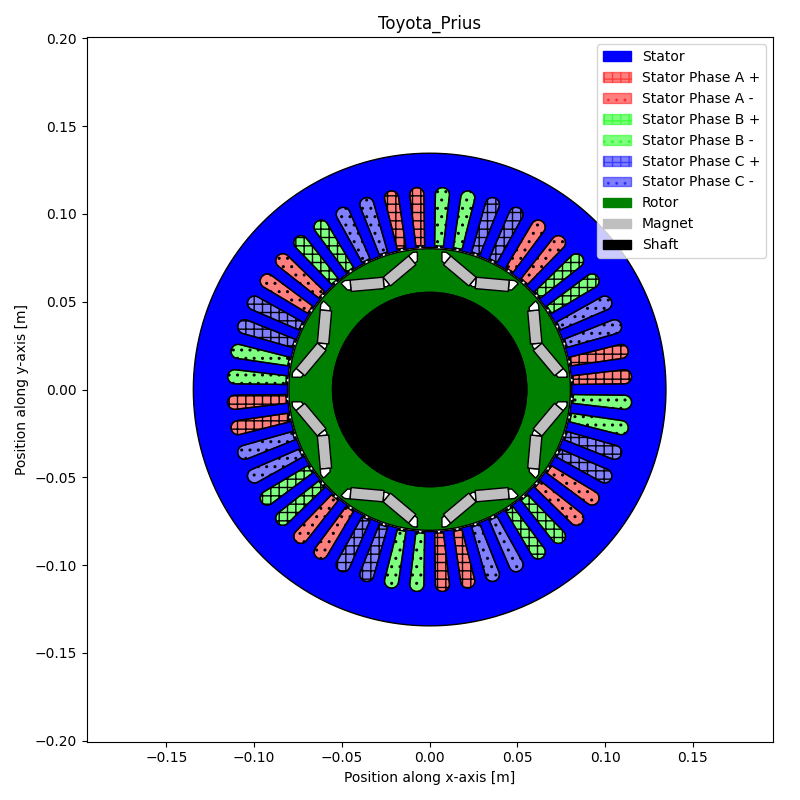:

(<Figure size 800x800 with 1 Axes>,
<AxesSubplot:title={'center':'Toyota_Prius'}, xlabel='Position along x-axis [m]', ylabel='Position along y-axis [m]'>)

:

from pyleecan.Classes.Simu1 import Simu1
from pyleecan.Classes.MagFEMM import MagFEMM
from pyleecan.Classes.InputCurrent import InputCurrent
from pyleecan.Classes.OPdq import OPdq
from numpy import sqrt, pi
# Initialization of the Simulation
simu_ref = Simu1(name="tuto_multi", machine=Toyota_Prius)

# Definition of the magnetic simulation (FEMM with symmetry and sliding band)
simu_ref.mag = MagFEMM(
type_BH_stator=0,
type_BH_rotor=0,
is_periodicity_a=True,
is_periodicity_t=True,
nb_worker=4,
Kgeo_fineness=1,
)
# Run only Magnetic module
simu_ref.elec = None
simu_ref.force = None
simu_ref.struct = None

simu_ref.input = InputCurrent()
simu_ref.input.Nt_tot = 128 # Number of time step
simu_ref.input.Na_tot = 2048 # Spatial discretization
# Set reference simulation operating point
simu_ref.input.OP = OPdq(N0 = 2000) # Rotor speed [rpm]
simu_ref.input.OP.Tem_av_ref = 353
simu_ref.input.OP.set_I0_Phi0(I0=250/sqrt(2), Phi0=140*pi/180)


The reference simulation “simu_ref” is now fully defined. It can be run before the multi-simulation to make sure that everything is correctly defined.

The multi-simulation will copy/update the reference simulation and its results. Thus, only the minimum input/output should be modified to save memory and computation time.

The reference simulation outputs are used for comparison purpose.

:

output_ref = simu_ref.run()

[17:17:59] Starting running simulation tuto_multi (machine=Toyota_Prius)
[17:17:59] Starting Magnetic module
[17:18:00] Computing Airgap Flux in FEMM
[17:18:09] End of simulation tuto_multi


In this tutorial we will focus on the main magnetic outputs. For example, after the reference simulation:

:

# Flux

# Torque
output_ref.mag.Tem.plot_2D_Data("time")
print("Main torque Output:")
print("Average Electromagnetic torque [N.m]:" +str(output_ref.mag.Tem_av))
print("Peak to Peak Torque ripple [N.m]:" +str(output_ref.mag.Tem_rip_pp))
print("Peak to Peak Torque ripple normalized according to average torque [-]:" +str(output_ref.mag.Tem_rip_norm))
print("Torque values (with symmetry):\n"+str(output_ref.mag.Tem.values))

# Operating point
print("\nOperating Point:")
print("Rotor speed [rpm]:"+str(output_ref.elec.OP.N0))
print("Id [Arms]:"+str(output_ref.elec.OP.Id_ref))
print("Iq [Arms]:"+str(output_ref.elec.OP.Iq_ref))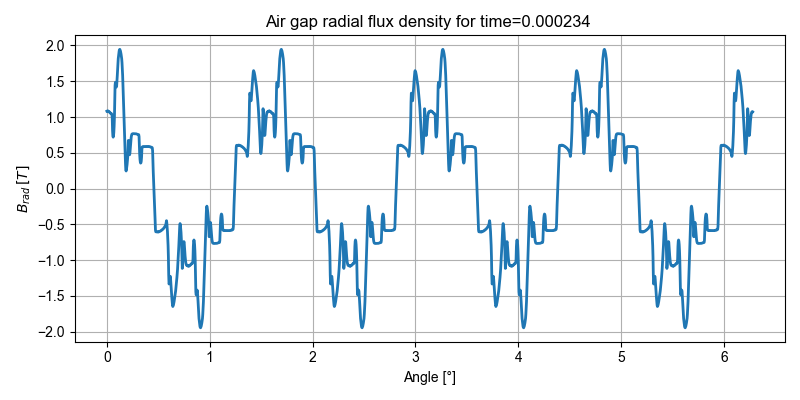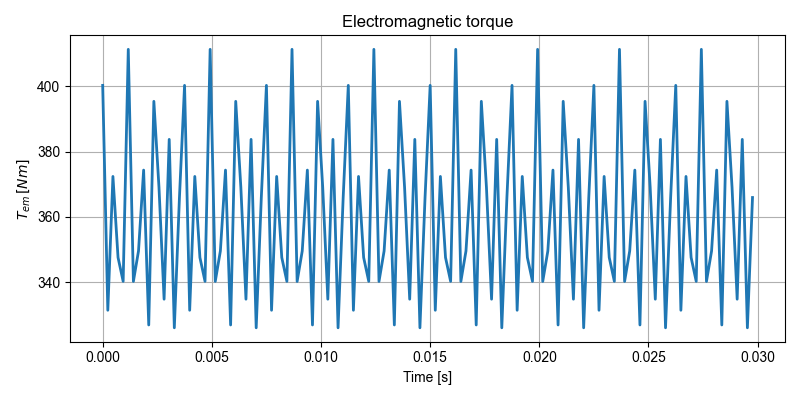Main torque Output:
Average Electromagnetic torque [N.m]:360.6008751685237
Peak to Peak Torque ripple [N.m]:85.14729536877104
Peak to Peak Torque ripple normalized according to average torque [-]:0.23612614730615444
Torque values (with symmetry):
[400.24401909 331.47882358 372.39270218 347.61076767 340.31963689
411.26212467 340.28986012 349.61059883 374.3362427  326.98128015
395.36026119 369.07272321 334.86255487 383.72566552 326.1148293
365.95191273]

Operating Point:
Rotor speed [rpm]:2000
Id [Arms]:-135.4188051049254
Iq [Arms]:113.62986941801093


Although the operation point was defined with I0/Phi0, pyleecan store and use only Id/Iq.

## First multi-simulation layer: Variable speed¶

The next step is to define the variable speed simulation. This tutorial uses the results from: Z. Yang, M. Krishnamurthy and I. P. Brown, “Electromagnetic and vibrational characteristic of IPM over full torque-speed range,” 2013 International Electric Machines & Drives Conference, Chicago, IL, 2013, pp. 295-302, doi: 10.1109/IEMDC.2013.6556267.

Each pyleecan simulation is assumed to be quasi-static and run on a single operating point (fixed speed). To run a simulation on several operating points two steps are needed: - First define a simulation that run correctly on a single operating point (like the one defined above), - then define a VarLoadCurrent object.

The VarLoadCurrent object is defined with a matrix with each line corresponding to an operating point (so each line will run a single speed simulation). The matrix columns order/containt are set by the user during the call to the method set_OP_matrix for more flexibility. Usually the first 3 columns are (N0, Id, Iq) or (N0 ,I0 ,Phi0)

A torque column can be added by adding “Tem” as a column name. It enables to define the reference torque for the Operating Point. The reference is stored in output.elec.Tem_av_ref, the real computed torque is stored in output.mag.Tem_av.

The following VarLoadCurrent object will run the previous simulation N_simu times by changing the value of Phi0.

Reference torque and current angle vector are:

:

from numpy import linspace, array, pi

Tem_av_ref = array([79, 125, 160, 192, 237, 281, 319, 343, 353, 332, 266, 164, 22]) # Yang et al, 2013
Phi0_ref = linspace(60 * pi / 180, 180 * pi / 180, Tem_av_ref.size)
N_speed = Tem_av_ref.size

:

from pyleecan.Classes.VarLoadCurrent import VarLoadCurrent
from numpy import zeros, ones, linspace, array, sqrt, arange

simu_vop = simu_ref.copy()
simu_vop.mag.import_file = None

# Creating the Operating point matrix
OP_matrix = zeros((N_speed,4))

# Set N0 = 2000 [rpm] for all simulation
OP_matrix[:,0] = 2000 * ones((N_speed))

# Set I0 = 250 / sqrt(2) [A] (RMS) for all simulation
OP_matrix[:,1] = 250/sqrt(2) * ones((N_speed))

# Set Phi0 from 60° to 180°
OP_matrix[:,2] = Phi0_ref

# Set reference torque from Yang et al, 2013
OP_matrix[:,3] = Tem_av_ref

print("Operating Point matrix:")
print(OP_matrix)
print("Original Operating point:")
print(simu_vop.input.OP.get_I0_Phi0())

# Column name are given in the order of OP_matrix

# The operating point of the simulation is automatically set as
# the first one from the OP_matrix
print("Updated Operating point:")
print(simu_vop.input.OP.get_I0_Phi0())

# All the simulation use the same machine
# No need to draw the machine for all OP

Operating Point matrix:
[[2.00000000e+03 1.76776695e+02 1.04719755e+00 7.90000000e+01]
[2.00000000e+03 1.76776695e+02 1.22173048e+00 1.25000000e+02]
[2.00000000e+03 1.76776695e+02 1.39626340e+00 1.60000000e+02]
[2.00000000e+03 1.76776695e+02 1.57079633e+00 1.92000000e+02]
[2.00000000e+03 1.76776695e+02 1.74532925e+00 2.37000000e+02]
[2.00000000e+03 1.76776695e+02 1.91986218e+00 2.81000000e+02]
[2.00000000e+03 1.76776695e+02 2.09439510e+00 3.19000000e+02]
[2.00000000e+03 1.76776695e+02 2.26892803e+00 3.43000000e+02]
[2.00000000e+03 1.76776695e+02 2.44346095e+00 3.53000000e+02]
[2.00000000e+03 1.76776695e+02 2.61799388e+00 3.32000000e+02]
[2.00000000e+03 1.76776695e+02 2.79252680e+00 2.66000000e+02]
[2.00000000e+03 1.76776695e+02 2.96705973e+00 1.64000000e+02]
[2.00000000e+03 1.76776695e+02 3.14159265e+00 2.20000000e+01]]
Original Operating point:
{'I0': 176.77669529663686, 'Phi0': 2.443460952792061}
Updated Operating point:
{'I0': 176.77669529663686, 'Phi0': 1.0471975511965976}


This first layer will behave as follow: - First the reference simulation will be run to get the reference output, - then the reference simulation will be duplicated N_speed times with the value of InputCurrent updated according to the OP matrix. - is_reuse_femm will update the N_speed simulations to reuse the FEMM file drawn in the reference simulation to speed-up the computations. - Each simulation of the list will be run separately and the results gathered in an XOutput object.

Pyleecan can detect that a simulation from the list matches the reference one and skip its computation to avoid computing twice the same thing. When defining a multi-simulation of multi-simulation, this optimization is essential since pyleecan will run “1 + (N_speed-1)” simulations instead of “1 + N_speed” for each combinaison of the design parameters. This is why the call to set_OP_matrix has overwrite the OP of the original simulation by the first OP of the matrix to make sure that we are in the “1 + (N_speed -1)” case. set_OP_matrix has two optional argument: - To disable this behavior “is_update_input=False” - To select another OP, “input_index=X” with X the matrix line number to use

To further understand pyleecan multi-simulation tools, this tutorial uses the option “is_keep_all_output” that keeps all the output of all the simulations. This option enables to access the plot for all the Operating Points but can take significant memory space depending on the simulation.

:

simu_vop.var_simu.is_keep_all_output = True

# Add I0/Phi0 to the data available at the end of the simulation
from pyleecan.Classes.DataKeeper import DataKeeper
I0_dk = DataKeeper(
name="Stator current rms amplitude",
symbol="I0",
unit="Arms",
keeper="lambda output: output.elec.OP.get_I0_Phi0()['I0']",
)
Phi0_dk = DataKeeper(
name="Stator current phase",
symbol="Phi0",
keeper="lambda output: output.elec.OP.get_I0_Phi0()['Phi0']",
)

:

Xout_vop = simu_vop.run()

[17:18:09] Starting running simulation tuto_multi (machine=Toyota_Prius)
[17:18:09] Computing reference simulation for Variable Load
[17:18:09] Starting Magnetic module
[17:18:11] Computing Airgap Flux in FEMM
[17:18:20] Variable Load Reference Results: I0=176.8 [Arms], Phi0=1.047 [rad], N0=2000 [rpm], Id=88.39 [Arms], Iq=153.1 [Arms], Tem_av_ref=79 [N.m], Tem_av=90.37 [N.m], Tem_rip_pp=30.72 [N.m], Tem_rip_norm=0.34 [-], Pem_av=1.893e+04 [W]
[===                                               ]   7%
[17:18:20] Variable Load: Using same FEMM file for all simulations (D:\GitHub\pyleecan-public\pyleecan\Results\tuto_multi\Femm\Toyota_Prius_model.fem)
[17:18:20] Running simulation 1/13 with N0=2000 [rpm], Id=88.39 [Arms], Iq=153.1 [Arms]
[17:18:20] Simulation matches reference one: Skipping computation
[17:18:20] Variable Load Results: I0=176.8 [Arms], Phi0=1.047 [rad], N0=2000 [rpm], Id=88.39 [Arms], Iq=153.1 [Arms], Tem_av_ref=79 [N.m], Tem_av=90.37 [N.m], Tem_rip_pp=30.72 [N.m], Tem_rip_norm=0.34 [-], Pem_av=1.893e+04 [W]
[=======                                           ]  14%
[17:18:20] Running simulation 2/13 with N0=2000 [rpm], Id=60.46 [Arms], Iq=166.1 [Arms]
[17:18:20] Starting Magnetic module
[17:18:23] Computing Airgap Flux in FEMM
[17:18:34] Variable Load Results: I0=176.8 [Arms], Phi0=1.222 [rad], N0=2000 [rpm], Id=60.46 [Arms], Iq=166.1 [Arms], Tem_av_ref=125 [N.m], Tem_av=134.1 [N.m], Tem_rip_pp=47.59 [N.m], Tem_rip_norm=0.3549 [-], Pem_av=2.808e+04 [W]
[==========                                        ]  21%
[17:18:34] Running simulation 3/13 with N0=2000 [rpm], Id=30.7 [Arms], Iq=174.1 [Arms]
[17:18:34] Starting Magnetic module
[17:18:36] Computing Airgap Flux in FEMM
[17:18:51] Variable Load Results: I0=176.8 [Arms], Phi0=1.396 [rad], N0=2000 [rpm], Id=30.7 [Arms], Iq=174.1 [Arms], Tem_av_ref=160 [N.m], Tem_av=179 [N.m], Tem_rip_pp=57.22 [N.m], Tem_rip_norm=0.3197 [-], Pem_av=3.748e+04 [W]
[==============                                    ]  28%
[17:18:51] Running simulation 4/13 with N0=2000 [rpm], Id=1.082e-14 [Arms], Iq=176.8 [Arms]
[17:18:51] Starting Magnetic module
[17:18:52] Computing Airgap Flux in FEMM
[17:19:01] Variable Load Results: I0=176.8 [Arms], Phi0=1.571 [rad], N0=2000 [rpm], Id=1.082e-14 [Arms], Iq=176.8 [Arms], Tem_av_ref=192 [N.m], Tem_av=223 [N.m], Tem_rip_pp=55.5 [N.m], Tem_rip_norm=0.2489 [-], Pem_av=4.669e+04 [W]
[=================                                 ]  35%
[17:19:01] Running simulation 5/13 with N0=2000 [rpm], Id=-30.7 [Arms], Iq=174.1 [Arms]
[17:19:01] Starting Magnetic module
[17:19:02] Computing Airgap Flux in FEMM
[17:19:10] Variable Load Results: I0=176.8 [Arms], Phi0=1.745 [rad], N0=2000 [rpm], Id=-30.7 [Arms], Iq=174.1 [Arms], Tem_av_ref=237 [N.m], Tem_av=264.2 [N.m], Tem_rip_pp=58.8 [N.m], Tem_rip_norm=0.2226 [-], Pem_av=5.533e+04 [W]
[=====================                             ]  42%
[17:19:10] Running simulation 6/13 with N0=2000 [rpm], Id=-60.46 [Arms], Iq=166.1 [Arms]
[17:19:10] Starting Magnetic module
[17:19:11] Computing Airgap Flux in FEMM
[17:19:20] Variable Load Results: I0=176.8 [Arms], Phi0=1.92 [rad], N0=2000 [rpm], Id=-60.46 [Arms], Iq=166.1 [Arms], Tem_av_ref=281 [N.m], Tem_av=301 [N.m], Tem_rip_pp=73.05 [N.m], Tem_rip_norm=0.2427 [-], Pem_av=6.303e+04 [W]
[=========================                         ]  50%
[17:19:20] Running simulation 7/13 with N0=2000 [rpm], Id=-88.39 [Arms], Iq=153.1 [Arms]
[17:19:20] Starting Magnetic module
[17:19:21] Computing Airgap Flux in FEMM
[17:19:32] Variable Load Results: I0=176.8 [Arms], Phi0=2.094 [rad], N0=2000 [rpm], Id=-88.39 [Arms], Iq=153.1 [Arms], Tem_av_ref=319 [N.m], Tem_av=331.3 [N.m], Tem_rip_pp=82.32 [N.m], Tem_rip_norm=0.2484 [-], Pem_av=6.939e+04 [W]
[============================                      ]  57%
[17:19:32] Running simulation 8/13 with N0=2000 [rpm], Id=-113.6 [Arms], Iq=135.4 [Arms]
[17:19:32] Starting Magnetic module
[17:19:32] Computing Airgap Flux in FEMM
[17:19:42] Variable Load Results: I0=176.8 [Arms], Phi0=2.269 [rad], N0=2000 [rpm], Id=-113.6 [Arms], Iq=135.4 [Arms], Tem_av_ref=343 [N.m], Tem_av=352.7 [N.m], Tem_rip_pp=85.22 [N.m], Tem_rip_norm=0.2416 [-], Pem_av=7.388e+04 [W]
[================================                  ]  64%
[17:19:42] Running simulation 9/13 with N0=2000 [rpm], Id=-135.4 [Arms], Iq=113.6 [Arms]
[17:19:42] Starting Magnetic module
[17:19:43] Computing Airgap Flux in FEMM
[17:19:51] Variable Load Results: I0=176.8 [Arms], Phi0=2.443 [rad], N0=2000 [rpm], Id=-135.4 [Arms], Iq=113.6 [Arms], Tem_av_ref=353 [N.m], Tem_av=360.6 [N.m], Tem_rip_pp=85.15 [N.m], Tem_rip_norm=0.2361 [-], Pem_av=7.552e+04 [W]
[===================================               ]  71%
[17:19:51] Running simulation 10/13 with N0=2000 [rpm], Id=-153.1 [Arms], Iq=88.39 [Arms]
[17:19:51] Starting Magnetic module
[17:19:53] Computing Airgap Flux in FEMM
[17:20:04] Variable Load Results: I0=176.8 [Arms], Phi0=2.618 [rad], N0=2000 [rpm], Id=-153.1 [Arms], Iq=88.39 [Arms], Tem_av_ref=332 [N.m], Tem_av=343.5 [N.m], Tem_rip_pp=67.67 [N.m], Tem_rip_norm=0.197 [-], Pem_av=7.193e+04 [W]
[=======================================           ]  78%
[17:20:04] Running simulation 11/13 with N0=2000 [rpm], Id=-166.1 [Arms], Iq=60.46 [Arms]
[17:20:04] Starting Magnetic module
[17:20:04] Computing Airgap Flux in FEMM
[17:20:14] Variable Load Results: I0=176.8 [Arms], Phi0=2.793 [rad], N0=2000 [rpm], Id=-166.1 [Arms], Iq=60.46 [Arms], Tem_av_ref=266 [N.m], Tem_av=276.6 [N.m], Tem_rip_pp=38.65 [N.m], Tem_rip_norm=0.1397 [-], Pem_av=5.794e+04 [W]
[==========================================        ]  85%
[17:20:14] Running simulation 12/13 with N0=2000 [rpm], Id=-174.1 [Arms], Iq=30.7 [Arms]
[17:20:14] Starting Magnetic module
[17:20:15] Computing Airgap Flux in FEMM
[17:20:25] Variable Load Results: I0=176.8 [Arms], Phi0=2.967 [rad], N0=2000 [rpm], Id=-174.1 [Arms], Iq=30.7 [Arms], Tem_av_ref=164 [N.m], Tem_av=157 [N.m], Tem_rip_pp=47.61 [N.m], Tem_rip_norm=0.3032 [-], Pem_av=3.289e+04 [W]
[==============================================    ]  92%
[17:20:25] Running simulation 13/13 with N0=2000 [rpm], Id=-176.8 [Arms], Iq=2.165e-14 [Arms]
[17:20:25] Starting Magnetic module
[17:20:26] Computing Airgap Flux in FEMM
[17:20:36] Variable Load Results: I0=176.8 [Arms], Phi0=3.142 [rad], N0=2000 [rpm], Id=-176.8 [Arms], Iq=2.165e-14 [Arms], Tem_av_ref=22 [N.m], Tem_av=-0.4102 [N.m], Tem_rip_pp=39.42 [N.m], Tem_rip_norm=-96.1 [-], Pem_av=-85.92 [W]
[==================================================] 100%
[17:20:36] End of simulation tuto_multi


The log of the simulation enables to understand step by step the status of the computation. As expected, the reference simulation is computed first. Then each of the 13 simulations is run starting with a message indicating the simulation operating point:

Running simulation 3/13 with N0=2000 [rpm], Id=30.7 [Arms], Iq=174.1 [Arms]

And ending with the Datakeepers result for this simulation:

Variable Load Results: N0=2000 [rpm], Id=30.7 [Arms], Iq=174.1 [Arms], I0=176.8 [A], Phi0=1.396 [], Tem_av_ref=160 [N.m], Tem_av=170.3 [N.m], Tem_rip_pp=53.12 [N.m], Tem_rip_norm=0.312 [-]

As expected the computation for the first simulation was skipped, the operating point and Datakeepers are still displayed as a reminder.

Pyleecan will automatically defines several Datakeeper to extract the main outputs from each simulation, we have manually added I0 and Phi0. These values are all gathered in the xoutput_dict:

:

print("Values available in XOutput:")
print(Xout_vop.xoutput_dict.keys())

print("\nI0 for each simulation:")
print(Xout_vop["I0"].result)
print("\nPhi0 for each simulation:")
print(Xout_vop["Phi0"].result)
print("\nComputed Average Torque for each simulation:")
print(Xout_vop["Tem_av"].result)
print("\nComputed Average Torque of the reference simulation:")
print(Xout_vop["Tem_av"].result_ref)

Values available in XOutput:
dict_keys(['I0', 'Phi0', 'N0', 'Id', 'Iq', 'Tem_av_ref', 'Tem_av', 'Tem_rip_pp', 'Tem_rip_norm', 'Pem_av'])

I0 for each simulation:
[176.77669529663686, 176.77669529663686, 176.77669529663683, 176.77669529663686, 176.77669529663683, 176.77669529663686, 176.77669529663686, 176.77669529663686, 176.77669529663686, 176.77669529663686, 176.77669529663683, 176.77669529663683, 176.77669529663686]

Phi0 for each simulation:
[1.0471975511965976, 1.2217304763960306, 1.3962634015954636, 1.5707963267948966, 1.7453292519943295, 1.9198621771937625, 2.0943951023931957, 2.2689280275926285, 2.443460952792061, 2.6179938779914944, 2.7925268031909276, 2.9670597283903604, 3.141592653589793]

Computed Average Torque for each simulation:
[90.3709250122835, 134.07947199821788, 178.95317285731952, 222.95208815546215, 264.1993260193586, 300.9664347929566, 331.328958899423, 352.7449529546559, 360.6008751685237, 343.4534568699727, 276.61962317091206, 157.02492548028775, -0.4102213992860144]

Computed Average Torque of the reference simulation:
90.3709250122835


As expected the average torque of the reference simulation is the same as the first simulation.

As the option is_keep_all_output was activated, one can also access all the simulation results in the “output_list”:

:

# Flux of the 3rd simulation
print("Id: "+str(Xout_vop.output_list.elec.OP.Id_ref)+" , Iq: "+str(Xout_vop.output_list.elec.OP.Iq_ref)+" , Tem_av_ref: "+str(Xout_vop.output_list.simu.input.OP.Tem_av_ref))
Xout_vop.output_list.mag.B.plot_2D_Data("angle")

print("Index of the reference simulation in the list: "+str(Xout_vop.xoutput_ref_index))
print("Id: "+str(Xout_vop.output_list.elec.OP.Id_ref)+" , Iq: "+str(Xout_vop.output_list.elec.OP.Iq_ref)+" , Tem_av_ref: "+str(Xout_vop.output_list.simu.input.OP.Tem_av_ref))
Xout_vop.output_list.mag.Tem.plot_2D_Data("time")

Id: 30.69695099224322 , Iq: 174.0910600800047 , Tem_av_ref: 160.0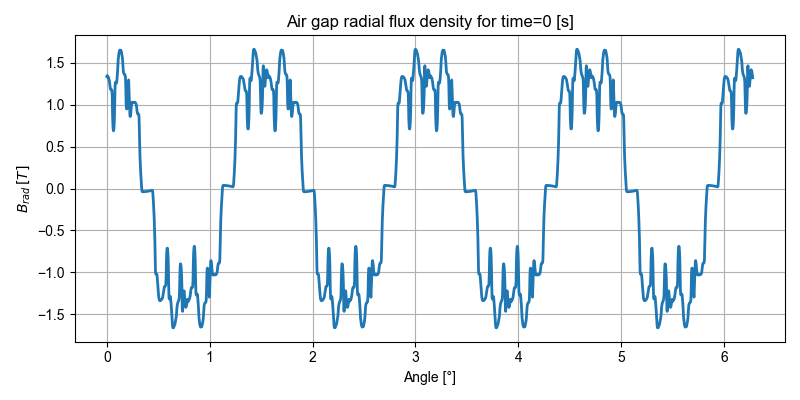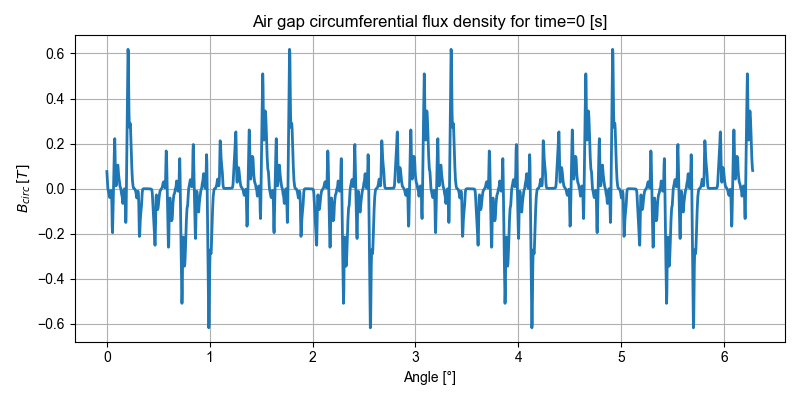Index of the reference simulation in the list: 0
Id: 88.38834764831844 , Iq: 153.0931089239486 , Tem_av_ref: 79.0